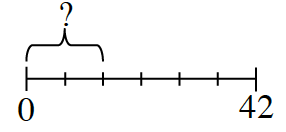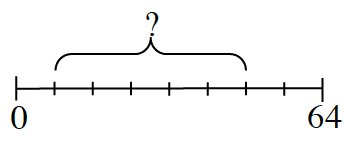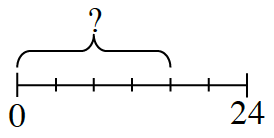### Home > CC2 > Chapter 4 > Lesson 4.1.1 > Problem4-9

4-9.

Find the missing parts of each number line. Assume that the lines have been split into equal parts.

1.Find the length of each segment. Then multiply that number by the number of segments in the missing part.

The segments are $7$ units long, and there are two segments in the missing part.

$14$

1.Use the method from part (a).

1.Use the method from part (a).